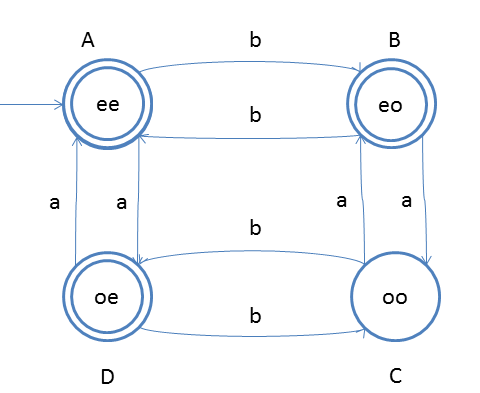# DFA String Examples

Design a DFA such a way that
Let string is w, Number of a(w) mod 2 = 0 or Number of b(w) mod 2 = 0. This means number of a should be divisible by 2 as well as number of b should also be divisible by 2.

If you see this question it just a bit variation of the above question.
The notations which we have used, we can choose which will be final state.
State NameState NotationFinal State
AeeYes
BeoYes
CooNo
DoeYesNote As the requirement is either number_of_a(w) mod 2 = 0 or number_of_b(w) mod 2=0 or both.
That is the reason we are assigning multiple final states.

### Testing

1. Take string ababbbb and traverse from left to right, here #a = 2 and #b = 5
2. from left first input is ‘a’ and start state ‘A’
3. so from state A we will go to state D
4. second input is ‘b’ and from D on b we will go to C
5. third input is ‘a’ and from C on a we will go state B
6. fourth input is ‘b’ and from B on b we will go to state A
7. fifth input is ‘b’ and from A on b we will go to state B
8. sixth input is ‘b’ and from B on b we will go to state A
9. seventh input is ‘b’ and from A we will go state B
After end of the string we are standing at state B, which is final state. So we say that string got accepted.